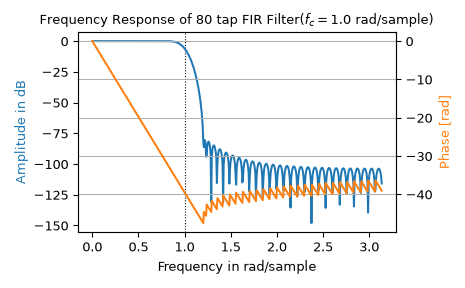# scipy.signal.freqz¶

scipy.signal.freqz(b, a=1, worN=512, whole=False, plot=None, fs=6.283185307179586, include_nyquist=False)[source]

Compute the frequency response of a digital filter.

Given the M-order numerator b and N-order denominator a of a digital filter, compute its frequency response:

```            jw                 -jw              -jwM
jw    B(e  )    b + be    + ... + b[M]e
H(e  ) = ------ = -----------------------------------
jw                 -jw              -jwN
A(e  )    a + ae    + ... + a[N]e
```
Parameters
barray_like

Numerator of a linear filter. If b has dimension greater than 1, it is assumed that the coefficients are stored in the first dimension, and `b.shape[1:]`, `a.shape[1:]`, and the shape of the frequencies array must be compatible for broadcasting.

aarray_like

Denominator of a linear filter. If b has dimension greater than 1, it is assumed that the coefficients are stored in the first dimension, and `b.shape[1:]`, `a.shape[1:]`, and the shape of the frequencies array must be compatible for broadcasting.

worN{None, int, array_like}, optional

If a single integer, then compute at that many frequencies (default is N=512). This is a convenient alternative to:

```np.linspace(0, fs if whole else fs/2, N, endpoint=include_nyquist)
```

Using a number that is fast for FFT computations can result in faster computations (see Notes).

If an array_like, compute the response at the frequencies given. These are in the same units as fs.

wholebool, optional

Normally, frequencies are computed from 0 to the Nyquist frequency, fs/2 (upper-half of unit-circle). If whole is True, compute frequencies from 0 to fs. Ignored if worN is array_like.

plotcallable

A callable that takes two arguments. If given, the return parameters w and h are passed to plot. Useful for plotting the frequency response inside `freqz`.

fsfloat, optional

The sampling frequency of the digital system. Defaults to 2*pi radians/sample (so w is from 0 to pi).

New in version 1.2.0.

include_nyquistbool, optional

If whole is False and worN is an integer, setting include_nyquist to True will include the last frequency (Nyquist frequency) and is otherwise ignored.

New in version 1.5.0.

Returns
wndarray

The frequencies at which h was computed, in the same units as fs. By default, w is normalized to the range [0, pi) (radians/sample).

hndarray

The frequency response, as complex numbers.

Notes

Using Matplotlib’s `matplotlib.pyplot.plot` function as the callable for plot produces unexpected results, as this plots the real part of the complex transfer function, not the magnitude. Try `lambda w, h: plot(w, np.abs(h))`.

A direct computation via (R)FFT is used to compute the frequency response when the following conditions are met:

1. An integer value is given for worN.

2. worN is fast to compute via FFT (i.e., `next_fast_len(worN)` equals worN).

3. The denominator coefficients are a single value (`a.shape == 1`).

4. worN is at least as long as the numerator coefficients (`worN >= b.shape`).

5. If `b.ndim > 1`, then `b.shape[-1] == 1`.

For long FIR filters, the FFT approach can have lower error and be much faster than the equivalent direct polynomial calculation.

Examples

```>>> from scipy import signal
>>> b = signal.firwin(80, 0.5, window=('kaiser', 8))
>>> w, h = signal.freqz(b)
```
```>>> import matplotlib.pyplot as plt
>>> fig, ax1 = plt.subplots()
>>> ax1.set_title('Digital filter frequency response')
```
```>>> ax1.plot(w, 20 * np.log10(abs(h)), 'b')
>>> ax1.set_ylabel('Amplitude [dB]', color='b')
```
```>>> ax2 = ax1.twinx()
>>> angles = np.unwrap(np.angle(h))
>>> ax2.plot(w, angles, 'g')
>>> ax2.grid()
>>> ax2.axis('tight')
>>> plt.show()
```Suppose we have two FIR filters whose coefficients are stored in the rows of an array with shape (2, 25). For this demonstration, we’ll use random data:

```>>> rng = np.random.default_rng()
>>> b = rng.random((2, 25))
```

To compute the frequency response for these two filters with one call to `freqz`, we must pass in `b.T`, because `freqz` expects the first axis to hold the coefficients. We must then extend the shape with a trivial dimension of length 1 to allow broadcasting with the array of frequencies. That is, we pass in `b.T[..., np.newaxis]`, which has shape (25, 2, 1):

```>>> w, h = signal.freqz(b.T[..., np.newaxis], worN=1024)
>>> w.shape
(1024,)
>>> h.shape
(2, 1024)
```

Now, suppose we have two transfer functions, with the same numerator coefficients `b = [0.5, 0.5]`. The coefficients for the two denominators are stored in the first dimension of the 2-D array a:

```a = [   1      1  ]
[ -0.25, -0.5 ]
```
```>>> b = np.array([0.5, 0.5])
>>> a = np.array([[1, 1], [-0.25, -0.5]])
```

Only a is more than 1-D. To make it compatible for broadcasting with the frequencies, we extend it with a trivial dimension in the call to `freqz`:

```>>> w, h = signal.freqz(b, a[..., np.newaxis], worN=1024)
>>> w.shape
(1024,)
>>> h.shape
(2, 1024)
```